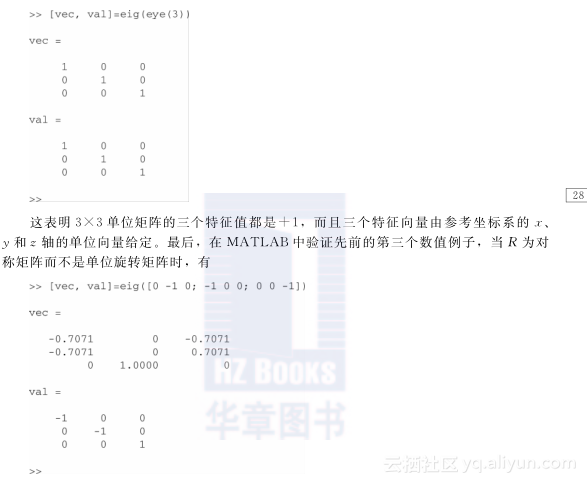《机器人与数字人：基于MATLAB的建模与控制》——2.3节指数映射和k过程

2.3指数映射和k过程

k2k3投影到给定坐标系中进行旋转，显然，‖k‖2=k21+k22+k23=1。给出其相应的斜对称矩阵为

``S(k)=K=0－k3k2k30－k1－k2k10显然，对这个3×3斜对称矩阵来说：1 K是斜对称矩阵，它的迹tr(K)=0；232 K2是对称矩阵，它的迹tr(K2)=-2；3 K3=-K。``

exp(K)=R∈SO(3)(27)

``exp(K)=I+K+22!K2+33!K3+44!K4+…=I+-33!+…K+22!-44!+…K2=I+sinK+(1-cos)K2=R(28)应用著名的泰勒展开式：sin=－33!+55!－…, cos=1－22!+44!－…假如给定坐标系绕单位向量k旋转角度，式(28)通常称为罗德里格斯公式，它提供了一种正向求解等效旋转矩阵R的方法。为了求逆解，首先，计算式(28)的迹，即tr(R)=3－2(1－cos)=1+2cos那么=arccostr(R)-12(29)因为RT=I－sinK+(1－cos)K2(210)将式(28)减去式(210)得R－RT=2sinK最后有K=R-RT2sin(211)``

``k=16121,K=160－1210－1－210因此，等效旋转矩阵为R=I+sin60°K+(1－cos60°)K2=05833-01869079040520208333-01869-062380520205833通过逆运算，可以应用上面的旋转矩阵R的逆解公式来确定角度和轴线k。根据方程(29)，由于tr(R)=2，=arccos(1/2)=60°，则有sin=3/2。那么，根据方程(211)，有K＝R-RT2sin=0-0408208165040820-04082-08165040820(212)``

``b=z10×z0=01－1－10－1110001=－1－10然后，选择单位向量b作为旋转轴k:k=b‖b‖=12－1－10， K=1200－10011－10为了求出基坐标z0轴相对于新坐标系z1轴绕k旋转的角度，可使用点积：zT0·z10=-1=‖z0‖‖z10‖cos=3cos那么cos=-13, sin=1－cos2=23注意到cos＜0，可见在第二象限，从而sin＞0。因此，可求得新坐标系的方向矩阵R10=I+sinK+(1－cos)K2=I+23K+1+13K2=0211307887－0577407887021130577405774－05774－05774此外，如果R是对称的，方程(211)将导致一个00不确定的极限。这意味着坐标系旋转角度如下：要么R=I，旋转0°；要么R≠I且RT=R，旋转180°。在第一种情况下R=I，由方程(29)计算=arccos(1)=0，可以略过公式(211)以避免出现奇异点和零点。然而，在第二种情况下，=arccos(-1)=180°，不能直接由方程(211)确定K。在这个特例中，sin=0，cos=-1。根据前面的方程(28)，R=I+sinK+(1-cos)K2=I+2K2，或者K2=12(R-I)（213）``

``R=0－10－10000－126R是对称矩阵，但R≠I，则tr(R)=-1，=180°。现在，令K=0－k3k2k30－k1－k2k10那么K2=-k23－k22k1k2k1k3k1k2－k23－k21k2k3k1k3k2k3－k21－k22因为R－I=－1－10－1－1000－2将上式与K2相比，根据式(213)我们首先得到非对角元素值k1k2=－12,k1k3=0，k2k3=0这意味着k3=0。然后，得到对角元素值k23+k22=k22=12,k23+k21=k21=12``

``k=2/2－2/20，=180°``

Rk=k+sinKk+(1－cos)K2k=k## 关于作者白红宇是个全栈工程师，前端vue，小程序，app开发到后端框架设计，数据库设计，环境部署上线运维。